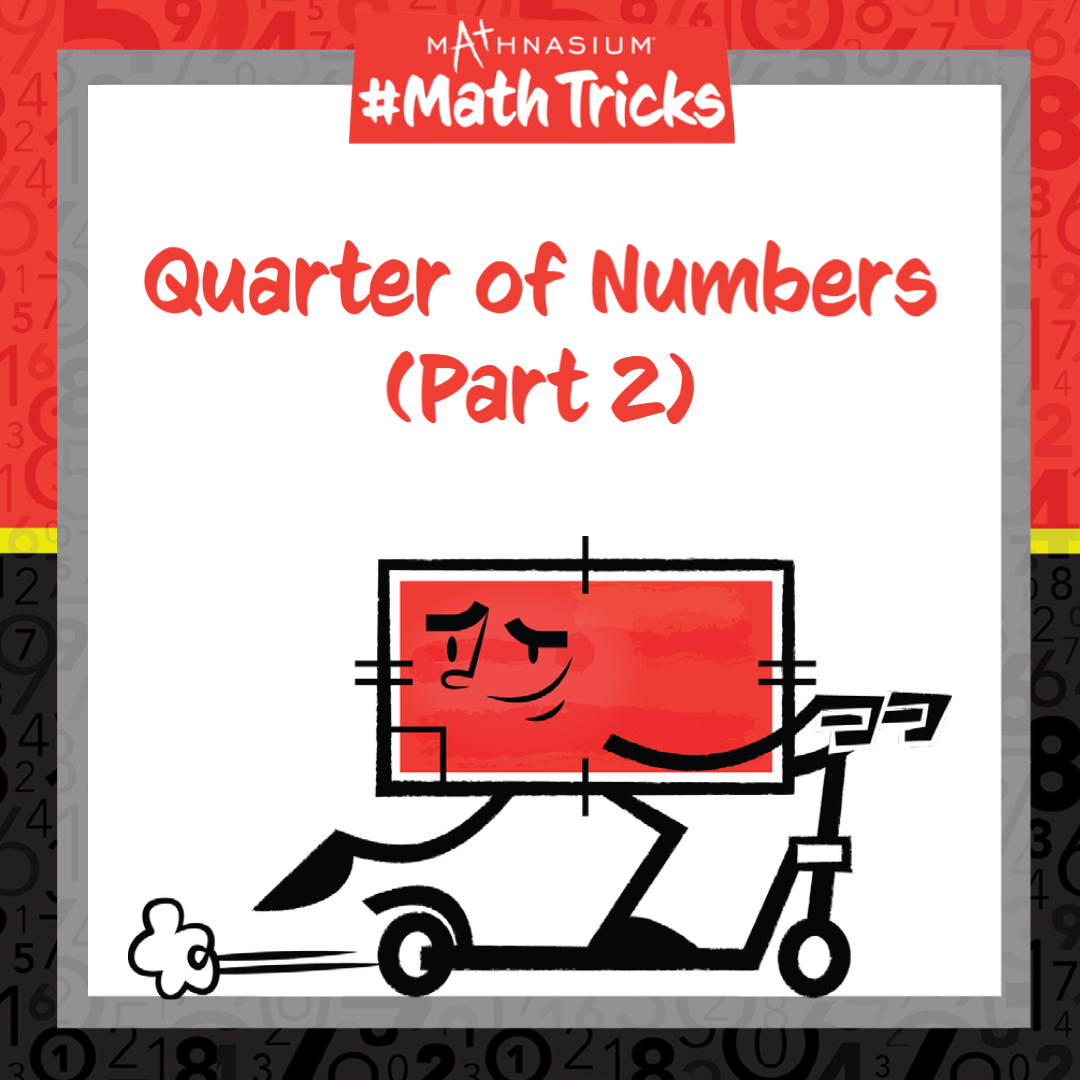877-601-6284
Get Started by Finding a Local Center

# Mathnasium #MathTricks: Quarter Of Numbers (Part 2)

Aug 3, 2022Welcome to Mathnasium’s Math Tricks series. Today we are sharing a trick to find a quarter of any number.

The word quarter means “four parts the same,” or “four equal parts.” To find a quarter of a number, we first cut the number in half and then cut it in half again, which means we find the half-of-a-half. Once we find one fourth of the whole, we can verify that the answer plus itself, doubled, is the original number.

Follow the example below to find the quarter amount.

#### Example: You are sharing a 15-pack of gum with three friends and yourself. How many pieces of gum do you get?

##### Step 1: Determine the problem to be solved.

Since you are sharing fifteen pieces of gum with four people, you will get a quarter of the whole amount.

##### Step 2: Find a half of the original amount.

Half of 15 is 7 ½.

##### Step 3: Find the half-of-the-half.

Half of 7 ½ is equal to half of 7 plus half of ½.

Half of 7 is equal to 3 ½.

Half of ½ is equal to ¼.

So, a quarter of 15 is equal to 3 ½ + ¼ = 3 ¾.

##### Step 4: Check the answer.

The answer plus itself is equal to 3 ¾ + 3 ¾ = 7 ½.

The result doubled is equal to 7 ½ x 2 = 15.

You, and each of your friends, will get 3 ¾ pieces of gum.

If you missed this, or any of our other Math Tricks videos, check them out on our YouTube channel!

## SEE HOW MATHNASIUM WORKS FOR YOUR SITUATION

### My Child is:## OUR METHOD WORKS

Mathnasium meets your child where they are and helps them with the customized program they need, for any level of mathematics.Early LearnersElementary SchoolMiddle SchoolHigh School